Open in App
Not now

# Decision Making in Java (if, if-else, switch, break, continue, jump)

• Difficulty Level : Easy
• Last Updated : 18 Feb, 2023

Decision Making in programming is similar to decision-making in real life. In programming also face some situations where we want a certain block of code to be executed when some condition is fulfilled.

A programming language uses control statements to control the flow of execution of a program based on certain conditions. These are used to cause the flow of execution to advance and branch based on changes to the state of a program.

Java’s Selection statements:

1. if: if statement is the most simple decision-making statement. It is used to decide whether a certain statement or block of statements will be executed or not i.e if a certain condition is true then a block of statements is executed otherwise not.

Syntax

```if(condition)
{
// Statements to execute if
// condition is true
}```

Here, the condition after evaluation will be either true or false. if statement accepts boolean values – if the value is true then it will execute the block of statements under it.
If we do not provide the curly braces ‘{‘ and ‘}’ after if( condition ) then by default if statement will consider the immediate one statement to be inside its block. For example,

```if(condition)  //Assume condition is true
statement1;  //part of if block
statement2;    // separate from if block

// Here if the condition is true
// if block will consider statement1 as its part and executes in only true condition
// statement2 will be separate from the if block so it will always executes whether the condition is true or false.```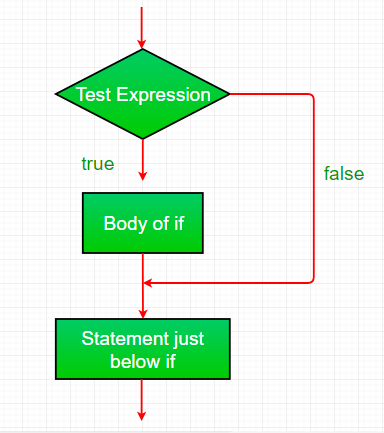Example:

## Java

 `// Java program to illustrate If statement without curly block` `import` `java.util.*;`   `class` `IfDemo {` `    ``public` `static` `void` `main(String args[])` `    ``{` `        ``int` `i = ``10``;`   `        ``if` `(i < ``15``)` `            ``System.out.println(``"Inside If block"``); ``// part of if block(immediate one statement after if condition)` `            ``System.out.println(``"10 is less than 15"``); ``//always executes as it is outside of if block` `        ``// This statement will be executed` `        ``// as if considers one statement by default again below statement is outside of if block` `        ``System.out.println(``"I am Not in if"``);` `    ``}` `}`

Output

```Inside If block
10 is less than 15
I am Not in if```

Time Complexity: O(1)
Auxiliary Space : O(1)

2. if-else: The if statement alone tells us that if a condition is true it will execute a block of statements and if the condition is false it won’t. But what if we want to do something else if the condition is false? Here comes the else statement. We can use the else statement with the if statement to execute a block of code when the condition is false.

Syntax

```if (condition)
{
// Executes this block if
// condition is true
}
else
{
// Executes this block if
// condition is false
}```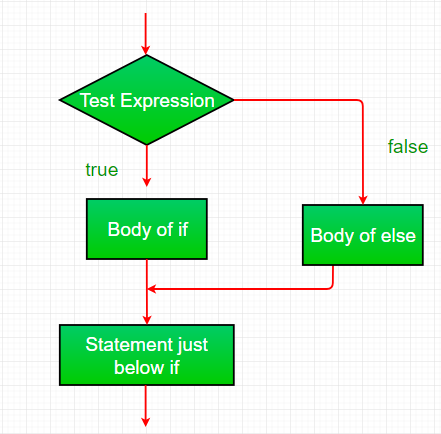Example:

## Java

 `// Java program to illustrate if-else statement` `import` `java.util.*;`   `class` `IfElseDemo {` `    ``public` `static` `void` `main(String args[])` `    ``{` `        ``int` `i = ``10``;`   `        ``if` `(i < ``15``)` `            ``System.out.println(``"i is smaller than 15"``);` `        ``else` `            ``System.out.println(``"i is greater than 15"``);` `    ``}` `}`

Output

`i is smaller than 15`

Time Complexity: O(1)
Auxiliary Space : O(1)

3. nested-if: A nested if is an if statement that is the target of another if or else. Nested if statements mean an if statement inside an if statement. Yes, java allows us to nest if statements within if statements. i.e, we can place an if statement inside another if statement.

Syntax:

```if (condition1)
{
// Executes when condition1 is true
if (condition2)
{
// Executes when condition2 is true
}
}```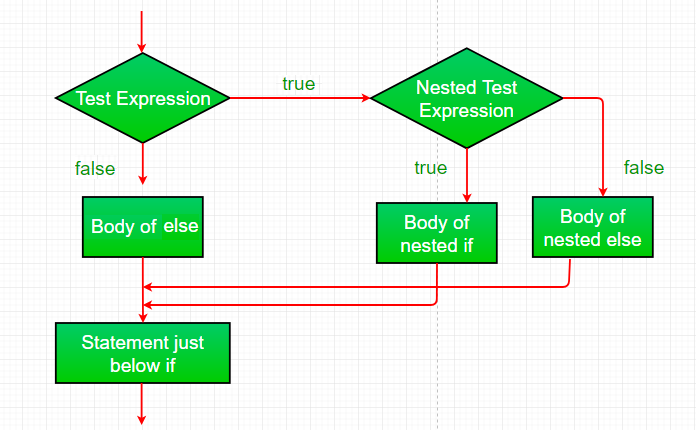Example:

## Java

 `// Java program to illustrate nested-if statement` `import` `java.util.*;`   `class` `NestedIfDemo {` `    ``public` `static` `void` `main(String args[])` `    ``{` `        ``int` `i = ``10``;`   `        ``if` `(i == ``10` `|| i<``15``) {` `            ``// First if statement` `            ``if` `(i < ``15``)` `                ``System.out.println(``"i is smaller than 15"``);`   `            ``// Nested - if statement` `            ``// Will only be executed if statement above` `            ``// it is true` `            ``if` `(i < ``12``)` `                ``System.out.println(` `                    ``"i is smaller than 12 too"``);` `        ``} ``else``{` `                ``System.out.println(``"i is greater than 15"``);` `        ``}` `    ``}` `}`

Output

```i is smaller than 15
i is smaller than 12 too```

Time Complexity: O(1)
Auxiliary Space: O(1)

4. if-else-if ladder: Here, a user can decide among multiple options.The if statements are executed from the top down. As soon as one of the conditions controlling the if is true, the statement associated with that ‘if’ is executed, and the rest of the ladder is bypassed. If none of the conditions is true, then the final else statement will be executed. There can be as many as ‘else if’ blocks associated with one ‘if’ block but only one ‘else’ block is allowed with one ‘if’ block.

```if (condition)
statement;
else if (condition)
statement;
.
.
else
statement;```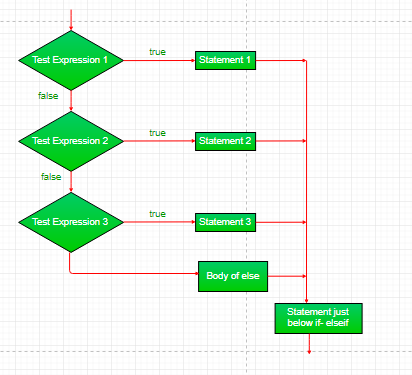Example:

## Java

 `// Java program to illustrate if-else-if ladder` `import` `java.util.*;`   `class` `ifelseifDemo {` `    ``public` `static` `void` `main(String args[])` `    ``{` `        ``int` `i = ``20``;`   `        ``if` `(i == ``10``)` `            ``System.out.println(``"i is 10"``);` `        ``else` `if` `(i == ``15``)` `            ``System.out.println(``"i is 15"``);` `        ``else` `if` `(i == ``20``)` `            ``System.out.println(``"i is 20"``);` `        ``else` `            ``System.out.println(``"i is not present"``);` `    ``}` `}`

Output

`i is 20`

Time Complexity: O(1)
Auxiliary Space: O(1)

5. switch-case: The switch statement is a multiway branch statement. It provides an easy way to dispatch execution to different parts of code based on the value of the expression.

Syntax:

```switch (expression)
{
case value1:
statement1;
break;
case value2:
statement2;
break;
.
.
case valueN:
statementN;
break;
default:
statementDefault;
}```

## Java

 `/*package whatever //do not write package name here */`   `import` `java.io.*;`   `class` `GFG {` `    ``public` `static` `void` `main (String[] args) {` `        ``int` `num=``20``;` `          ``switch``(num){` `          ``case` `5` `:  System.out.println(``"It is 5"``);` `                    ``break``;` `          ``case` `10` `: System.out.println(``"It is 10"``);` `                    ``break``;` `          ``case` `15` `: System.out.println(``"It is 15"``);` `                    ``break``;` `          ``case` `20` `: System.out.println(``"It is 20"``);` `                    ``break``;` `          ``default``:  System.out.println(``"Not present"``);` `            `  `        ``}` `    ``}` `}`

Output

```It is 20
```

Time Complexity: O(1)

Space Complexity: O(1)

• The expression can be of type byte, short, int char, or an enumeration. Beginning with JDK7, the expression can also be of type String.
• Duplicate case values are not allowed.
• The default statement is optional.
• The break statement is used inside the switch to terminate a statement sequence.
• The break statements are necessary without the break keyword, statements in switch blocks fall through.
• If the break keyword is omitted, execution will continue to the next case.

6. jump: Java supports three jump statements: break, continue and return. These three statements transfer control to another part of the program.

• Break: In Java, a break is majorly used for:
• Terminate a sequence in a switch statement (discussed above).
• To exit a loop.
• Used as a “civilized” form of goto.
• Continue: Sometimes it is useful to force an early iteration of a loop. That is, you might want to continue running the loop but stop processing the remainder of the code in its body for this particular iteration. This is, in effect, a goto just past the body of the loop, to the loop’s end. The continue statement performs such an action.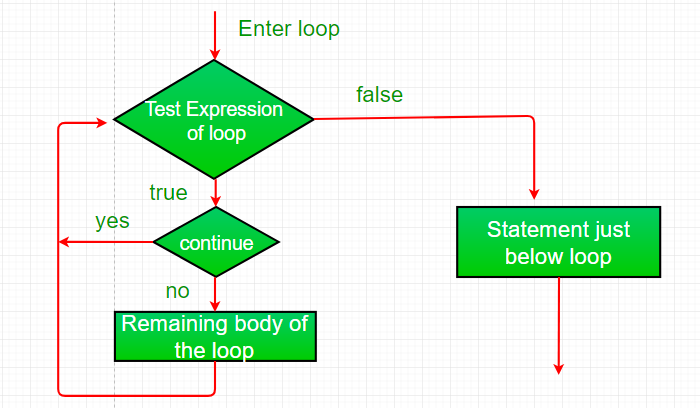Example:

## Java

 `// Java program to illustrate using` `// continue in an if statement` `import` `java.util.*;`   `class` `ContinueDemo {` `    ``public` `static` `void` `main(String args[])` `    ``{` `        ``for` `(``int` `i = ``0``; i < ``10``; i++) {` `            ``// If the number is even` `            ``// skip and continue` `            ``if` `(i % ``2` `== ``0``)` `                ``continue``;`   `            ``// If number is odd, print it` `            ``System.out.print(i + ``" "``);` `        ``}` `    ``}` `}`

Output

`1 3 5 7 9 `

Time Complexity: O(1)
Auxiliary Space: O(1)

• Return: The return statement is used to explicitly return from a method. That is, it causes program control to transfer back to the caller of the method.

Example:

## Java

 `// Java program to illustrate using return` `import` `java.util.*;`   `public` `class` `Return {` `    ``public` `static` `void` `main(String args[])` `    ``{` `        ``boolean` `t = ``true``;` `        ``System.out.println(``"Before the return."``);`   `        ``if` `(t)` `            ``return``;`   `        ``// Compiler will bypass every statement` `        ``// after return` `        ``System.out.println(``"This won't execute."``);` `    ``}` `}`

Output

`Before the return.`

Time Complexity: O(1)
Auxiliary Space: O(1)

This article is contributed by Anuj Chauhan and Harsh Aggarwal. If you like GeeksforGeeks and would like to contribute, you can also write an article using write.geeksforgeeeks.org or mail your article to review-team@geeksforgeeks.org. See your article appearing on the GeeksforGeeks main page and help other Geeks.